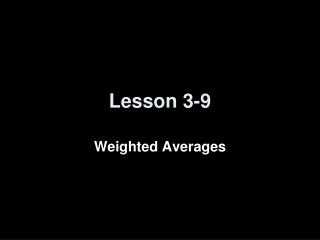Download PresentationLesson 3-9Lesson 3-9 - PowerPoint PPT Presentation

Download PresentationLesson 3-9
An Image/Link below is provided (as is) to download presentation

Download Policy: Content on the Website is provided to you AS IS for your information and personal use and may not be sold / licensed / shared on other websites without getting consent from its author. While downloading, if for some reason you are not able to download a presentation, the publisher may have deleted the file from their server.

- - - - - - - - - - - - - - - - - - - - - - - - - - - E N D - - - - - - - - - - - - - - - - - - - - - - - - - - -
Presentation Transcript

1. Lesson 3-9 Weighted Averages

2. Objectives • Solve mixture problems • Solve uniform motion problems

3. Vocabulary • Weighted average – sum of the product of the number of units and the value per unit divided by the sum of the number of units • Mixture problems – problems in which two or more parts are combined into a whole • Uniform motion problems – problems where an object moves at certain speed or rate

4. Mixture Problems • Step 1:

5. Example 1 PetsJeri likes to feed her cat gourmet cat food that costs \$1.75 per pound. However, food at that price is too expensive so she combines it with cheaper cat food that costs \$0.50 per pound. How many pounds of cheaper food should Jeri buy to go with 5 pounds of gourmet food, if she wants the price to be \$1.00 per pound? Let w = the number of pounds of cheaper cat food. Make a table. 5 \$1.75 \$8.75 w \$0.50 0.5w 5 + w \$1.00 1.00(5 + w)

6. Subtract 5.0 from each side. Simplify. Divide each side by 0.5. Original equation Simplify. Distributive Property Subtract 0.5w from each side. Simplify. Example 1 cont Answer: Jerry should buy 7.5 pounds of cheaper cat food to be mixed with the 4 pounds of gourmet cat food to equal out to \$1.00 per pound of cat food.

7. Amount of Solution (gallons) Price 35% Solution 100% Solution 50% Solution Example 2 Auto MaintenanceTo provide protection against freezing, a car’s radiator should contain a solution of 50% antifreeze. Darryl has 2 gallons of a 35% antifreeze solution. How many gallons of 100% antifreeze should Darryl add to his solution to produce a solution of 50% antifreeze? Let g = the number of gallons of 100% antifreeze to be added. Make a table. 2 0.35(2) g 1.0(g) 2 + g 0.50(2 + g)

8. Subtract 0.70 from each side. Amount of antifreeze in 35% solution plus amount of antifreeze in 100% solution equals amount of antifreeze in 50% solution. Simplify. 0.35(2) 1.0(g) 0.50(2 +g) Divide each side by 0.50. Original equation Distributive Property Simplify. Subtract 0.50g from each side. Simplify. Example 2 cont Answer:Darryl should add 0.60 gallons of 100% antifreeze to produce a 50% solution.

9. Air TravelMirasol took a non-stop flight from Newark to Austin to visit her grandmother. The 1500-mile trip took three hours and 45 minutes. Because of bad weather, the return trip took four hours and 45 minutes. What was her average speed for the round trip? Going Returning To find the average speed for each leg of the trip, rewrite . Example 3

10. Round Trip Definition of weighted average Simplify. Example 3 cont Answer:The average speed for the round trip was about 343.9 miles per hour.

11. Takes 5 miles to stop Takes 3 miles to stop Example 4 RescueA railroad switching operator has discovered that two trains are heading toward each other on the same track. Currently, the trains are 53 miles apart. One train is traveling at 75 miles per hour and the other train is traveling at 40 miles per hour. The faster train will require 5 miles to stop safely, and the slower train will require 3 miles to stop safely. About how many minutes does the operator have to warn the train engineers to stop their trains? Draw a diagram. 53 miles apart

12. Round to the nearest hundredth. Convert to minutes by multiplying by 60. d = rt r t Fast train Original equation Other train Simplify. Divide each side by 115. Example 4 cont Let m = the number of minutes that the operator has to warn the train engineers to stop their trains safely. Make a table. 75 m 75m 40 m 40m Answer:The operator has about 23 minutes to warn the engineers.

13. Summary & Homework • Summary: • The weighted average of a set of data is the sum of the product of each number in the set and its weight divided by the sum of all the weights • The formula d = rt (distance = rate of change (velocity)  time) is used to solve uniform motion problems • Homework: • none